# Calculus 2 : Derivatives of Vectors

## Example Questions

1 2 4 Next →

### Example Question #31 : Derivatives Of Vectors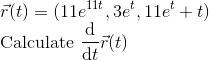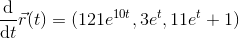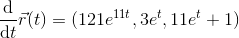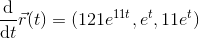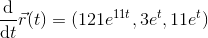Explanation:

In general:

If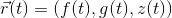,

then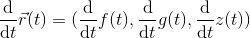Derivative rules that will be needed here:

• Taking a derivative on a term, or using the power rule, can be done by doing the following: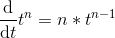• When taking derivatives of sums, evaluate with the sum rule which states that the derivative of the sum is the same as the sum of the derivative of each term: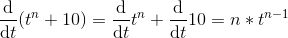• Special rule when differentiating an exponential function: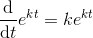, where k is a constant.

In this problem,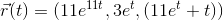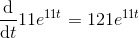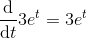Put it all together to get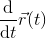### Example Question #501 : Parametric, Polar, And Vector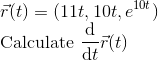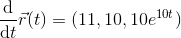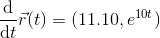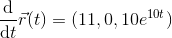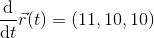Explanation:

In general:

If,

thenDerivative rules that will be needed here:

• Taking a derivative on a term, or using the power rule, can be done by doing the following:• Special rule when differentiating an exponential function:, where k is a constant.

In this problem,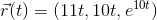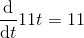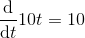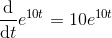Put it all together to get### Example Question #502 : Parametric, Polar, And Vector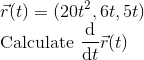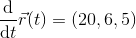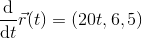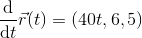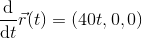Explanation:

In general:

If,

thenDerivative rules that will be needed here:

• Taking a derivative on a term, or using the power rule, can be done by doing the following:In this problem,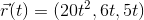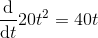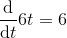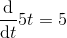Put it all together to get### Example Question #503 : Parametric, Polar, And Vector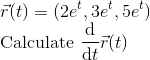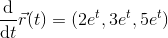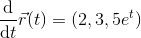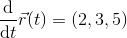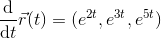Explanation:

In general:

If,

thenDerivative rules that will be needed here:

• Special rule when differentiating an exponential function:, where k is a constant.

In this problem,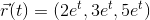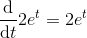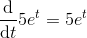Put it all together to get### Example Question #504 : Parametric, Polar, And Vector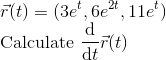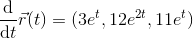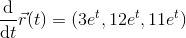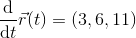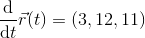Explanation:

In general:

If,

thenDerivative rules that will be needed here:

• Special rule when differentiating an exponential function:, where k is a constant.

In this problem,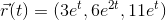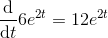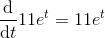Put it all together to get### Example Question #505 : Parametric, Polar, And Vector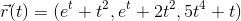Calculate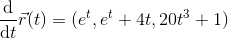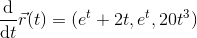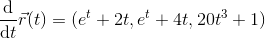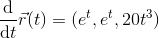Explanation:

In general:

If,

thenDerivative rules that will be needed here:

• Taking a derivative on a term, or using the power rule, can be done by doing the following:• When taking derivatives of sums, evaluate with the sum rule which states that the derivative of the sum is the same as the sum of the derivative of each term:• Special rule when differentiating an exponential function:, where k is a constant.

In this problem,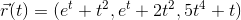Use the sum rule and the power rule on each of the components.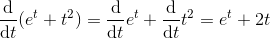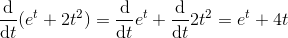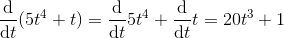Put it all together to get1 2 4 Next →